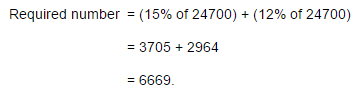Courses

# Test: Pie Chart- 3

## 5 Questions MCQ Test Integrated Reasoning for GMAT | Test: Pie Chart- 3

Description
This mock test of Test: Pie Chart- 3 for LR helps you for every LR entrance exam. This contains 5 Multiple Choice Questions for LR Test: Pie Chart- 3 (mcq) to study with solutions a complete question bank. The solved questions answers in this Test: Pie Chart- 3 quiz give you a good mix of easy questions and tough questions. LR students definitely take this Test: Pie Chart- 3 exercise for a better result in the exam. You can find other Test: Pie Chart- 3 extra questions, long questions & short questions for LR on EduRev as well by searching above.
QUESTION: 1

### The following pie-charts show the distribution of students of graduate and post-graduate levels in seven different institutes in a town.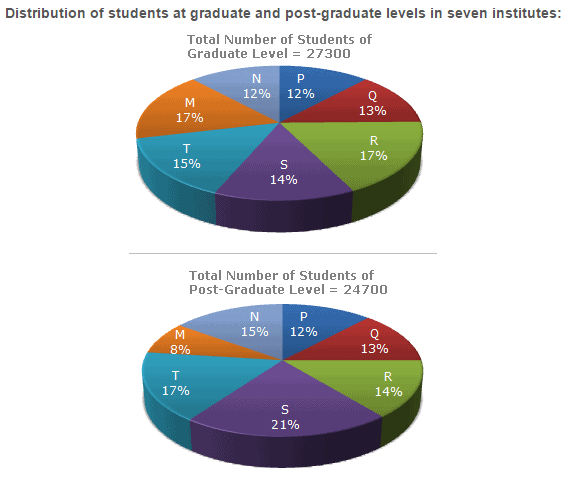What is the total number of graduate and post-graduate level students is institute R?

Solution: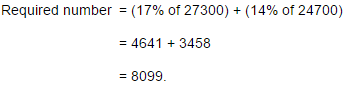QUESTION: 2

### The following pie-charts show the distribution of students of graduate and post-graduate levels in seven different institutes in a town.What is the ratio between the number of students studying at post-graduate and graduate levels respectively from institute S?

Solution: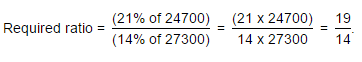QUESTION: 3

### The following pie-charts show the distribution of students of graduate and post-graduate levels in seven different institutes in a town.How many students of institutes of M and S are studying at graduate level?

Solution: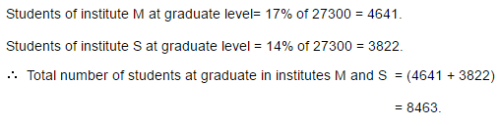QUESTION: 4

The following pie-charts show the distribution of students of graduate and post-graduate levels in seven different institutes in a town.What is the ratio between the number of students studying at post-graduate level from institutes S and the number of students studying at graduate level from institute Q?

Solution:QUESTION: 5

The following pie-charts show the distribution of students of graduate and post-graduate levels in seven different institutes in a town.Total number of students studying at post-graduate level from institutes N and P is

Solution: